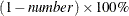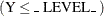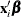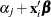OUTPUT Statement

OUTPUT <OUT=SAS-data-set> <keyword=name …keyword=name> ;

The OUTPUT statement creates a new SAS data set that contains all the variables in the input data set and, optionally, the estimated linear predictors (XBETA) and their standard error estimates, the weights for the Hessian matrix, predicted values of the mean, confidence limits for predicted values, residuals, and case deletion diagnostics. Residuals and diagnostic statistics are not computed for multinomial models.

You can also request these statistics with the OBSTATS, PREDICTED, RESIDUALS, DIAGNOSTICS | INFLUENCE, CL, or XVARS option in the MODEL statement. You can then create a SAS data set containing them with ODS OUTPUT commands.

You might prefer to specify the OUTPUT statement for requesting these statistics since the following are true:

• The OUTPUT statement produces no tabular output.

• The OUTPUT statement creates a SAS data set more efficiently than ODS. This can be an advantage for large data sets.

• You can specify the individual statistics to be included in the SAS data set.

If you use the multinomial distribution with one of the cumulative link functions for ordinal data, the data set also contains variables named _ORDER_ and _LEVEL_ that indicate the levels of the ordinal response variable and the values of the variable in the input data set corresponding to the sorted levels. These variables indicate that the predicted value for a given observation is the probability that the response variable is as large as the value of the _LEVEL_ variable. Residuals and other diagnostic statistics are not available for the multinomial distribution.

The estimated linear predictor, its standard error estimate, and the predicted values and their confidence intervals are computed for all observations in which the explanatory variables are all nonmissing, even if the response is missing. By adding observations with missing response values to the input data set, you can compute these statistics for new observations or for settings of the explanatory variables not present in the data without affecting the model fit.

The following list explains specifications in the OUTPUT statement.

OUT=SAS-data-set

specifies the output data set. If you omit the OUT=option, the output data set is created and given a default name that uses the DATAn convention.

keyword=name

specifies the statistics to be included in the output data set and names the new variables that contain the statistics. Specify a keyword for each desired statistic (see the following list of keywords), an equal sign, and the name of the new variable or variables to contain the statistic. You can list only one variable after the equal sign for all the statistics, except for the case deletion diagnostics for individual parameter estimates, DFBETA, DFBETAS, DFBETAC, and DFBETACS. You can list variables enclosed in parentheses to correspond to the variables in the model, or you can specify the keyword _all_, without parentheses, to include deletion diagnostics for all of the parameters in the model.

Although you can use the OUTPUT statement without any keyword=name specifications, the output data set then contains only the original variables and, possibly, the variables Level and Value (if you use the multinomial model with ordinal data). Note that the residuals and deletion diagnostics are not available for the multinomial model with ordinal data. Some of the case deletion diagnostic statistics apply only to models for correlated data specified with a REPEATED statement. If you request these statistics for ordinary generalized linear models, the values of the corresponding variables are set to missing in the output data set. Formulas for the statistics are given in the section Predicted Values of the Mean, the section Residuals, and the section Case Deletion Diagnostic Statistics.

The keywords allowed and the statistics they represent are as follows:

DFBETA | DBETA

represents the effect of deleting an observation on parameter estimates. If you specify the keyword _all_ after the equal sign, variables named DFBETA_ParameterName will be included in the output data set to contain the values of the diagnostic statistic to measure the influence of deleting a single observation on the individual parameter estimates. ParameterName is the name of the regression model parameter formed from the input variable names concatenated with the appropriate levels, if classification variables are involved.

DFBETAS | DBETAS

represents the effect of deleting an observation on standardized parameter estimates. If you specify the keyword _all_ after the equal sign, variables named DFBETAS_ParameterName will be included in the output data set to contain the values of the diagnostic statistic to measure the influence of deleting a single observation on the individual parameter estimates. ParameterName is the name of the regression model parameter formed from the input variable names concatenated with the appropriate levels, if classification variables are involved.

DOBS | COOKD | COOKSD

represents the Cook distance type statistic to measure the influence of deleting a single observation on the overall model fit.

HESSWGT

represents the diagonal element of the weight matrix used in computing the Hessian matrix.

H | LEVERAGE

represents the leverage of a single observation.

LOWER | L

represents the lower confidence limit for the predicted value of the mean, or the lower confidence limit for the probability that the response is less than or equal to the value of Level or Value. The confidence coefficient is determined by the ALPHA=number option in the MODEL statement as. The default confidence coefficient is 95%.

PREDICTED | PRED | PROB | P

represents the predicted value of the mean of the response or the predicted probability that the response variable is less than or equal to the value of _LEVEL_ if the multinomial model for ordinal data is used (in other words, Pr, where Y is the response variable).

PZERO

represents the zero-inflation probability for zero-inflated models.

RESCHI

represents the Pearson (chi) residual for identifying observations that are poorly accounted for by the model.

RESDEV

represents the deviance residual for identifying poorly fitted observations.

RESLIK

represents the likelihood residual for identifying poorly fitted observations.

RESRAW

represents the raw residual for identifying poorly fitted observations.

STDRESCHI

represents the standardized Pearson (chi) residual for identifying observations that are poorly accounted for by the model.

STDRESDEV

represents the standardized deviance residual for identifying poorly fitted observations.

STDXBETA

represents the standard error estimate of XBETA (see the XBETA keyword).

UPPER | U

represents the upper confidence limit for the predicted value of the mean, or the upper confidence limit for the probability that the response is less than or equal to the value of Level or Value. The confidence coefficient is determined by the ALPHA=number option in the MODEL statement as. The default confidence coefficient is 95%.

XBETA

represents the estimate of the linear predictorfor observation i, or, where j is the corresponding ordered value of the response variable for the multinomial model with ordinal data. If there is an offset, it is included in.

The keywords in the following list apply only to models specified with a REPEATED statement, fit by generalized estimating equations (GEEs).

CH | CLUSTERH | CLEVERAGE

represents the leverage of a cluster.

CLUSTER

represents the numerical cluster index, in order of sorted clusters.

DCLS | CLUSTERCOOKD | CLUSTERCOOKSD

represents the Cook distance type statistic to measure the influence of deleting an entire cluster on the overall model fit.

DFBETAC | DBETAC

represents the effect of deleting an entire cluster on parameter estimates. If you specify the keyword _all_ after the equal sign, variables named DFBETAC_ParameterName will be included in the output data set to contain the values of the diagnostic statistic to measure the influence of deleting the cluster on the individual parameter estimates. ParameterName is the name of the regression model parameter formed from the input variable names concatenated with the appropriate levels, if classification variables are involved.

DFBETACS | DBETACS

represents the effect of deleting an entire cluster on normalized parameter estimates. If you specify the keyword _all_ after the equal sign, variables named DFBETACS_ParameterName will be included in the output data set to contain the values of the diagnostic statistic to measure the influence of deleting the cluster on the individual parameter estimates, normalized by their standard errors. ParameterName is the name of the regression model parameter formed from the input variable names concatenated with the appropriate levels, if classification variables are involved.

MCLS | CLUSTERDFIT

represents the studentized Cook distance type statistic to measure the influence of deleting an entire cluster on the overall model fit.